# Convolution Calculation (piecewise function)

## Homework Statement

Compute the convolution ##(f*h)(t)## where

$$f(t) = \left\{\begin{matrix}1, \ \ for \ \ |t|<1 \\ 0, \ \ \ \ otherwise \end{matrix}\right.$$

and

$$h(t) = \left\{\begin{matrix}2|t|-1, \ \ for \ \ |t|<1/2 \\ 0, \ \ \ \ otherwise \end{matrix}\right.$$

## Homework Equations

Convolution integral: ##(f*h)(t)= \int^\infty_{-\infty} f(\tau) h(t-\tau) d \tau##

## The Attempt at a Solution

[/B]
Here I first made a sketch of the two functions: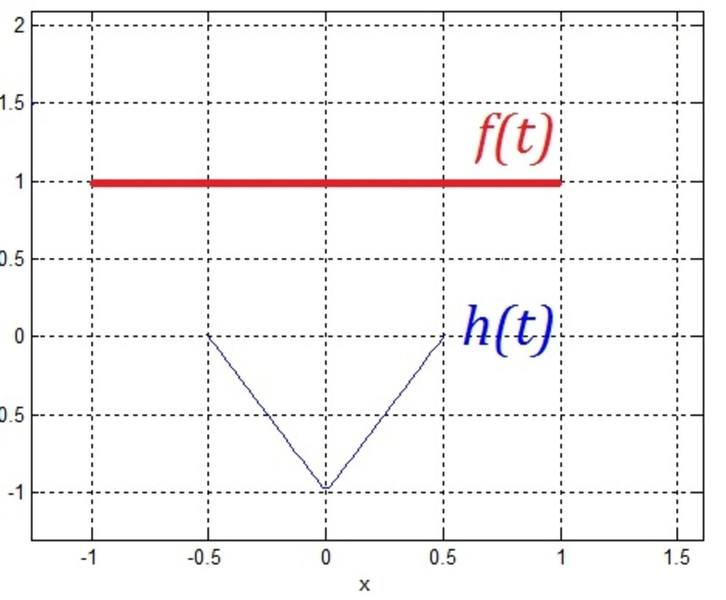f(t) is non-zero on [-1, 1] and g(t) is non-zero on [-0.5, 0.5], so adding these two we find that (f ∗ g)(t) should be nonzero on [-1.5, 1.5]. The list of change points of (f ∗ g)(t) is {-1.5, -0.5, 0.5, 1.5}. For values t that are outside this range the convolution is zero.

So, do I need to calculate the convolution for 3 separate cases?

I mean ##-1.5 \leq t \leq -0.5##, ##-0.5 \leq t \leq 0.5##, and ##0.5 \leq t \leq 1.5##?

Here is my attempt:

First case: we want to integrate from -1.5 to -0.5 using the formula given above (##(f*g)(t)=\int^{0.5}_{-1.5} f(\tau) h(t-\tau) d \tau##). ##h(t)## is zero. But for ##f(t)## the function is zero on the interval ##(-1.5, -1)##, whereas it is equal to 1 from ##(-1, -0.5)##, so what value do I need to be using for the function?Any help is greatly appreciated.

Samy_A
Homework Helper

## Homework Statement

Compute the convolution ##(f*h)(t)## where

$$f(t) = \left\{\begin{matrix}1, \ \ for \ \ |t|<1 \\ 0, \ \ \ \ otherwise \end{matrix}\right.$$

and

$$h(t) = \left\{\begin{matrix}2|t|-1, \ \ for \ \ |t|<1/2 \\ 0, \ \ \ \ otherwise \end{matrix}\right.$$

## Homework Equations

Convolution integral: ##(f*h)(t)= \int^\infty_{-\infty} f(\tau) h(t-\tau) d \tau##

## The Attempt at a Solution

[/B]
Here I first made a sketch of the two functions:f(t) is non-zero on [-1, 1] and g(t) is non-zero on [-0.5, 0.5], so adding these two we find that (f ∗ g)(t) should be nonzero on [-1.5, 1.5]. The list of change points of (f ∗ g)(t) is {-1.5, -0.5, 0.5, 1.5}. For values t that are outside this range the convolution is zero.

So, do I need to calculate the convolution for 3 separate cases?

I mean ##-1.5 \leq t \leq -0.5##, ##-0.5 \leq t \leq 0.5##, and ##0.5 \leq t \leq 1.5##?

Here is my attempt:

First case: we want to integrate from -1.5 to -0.5 using the formula given above (##(f*g)(t)=\int^{0.5}_{-1.5} f(\tau) h(t-\tau) d \tau##). ##h(t)## is zero. But for ##f(t)## the function is zero on the interval ##(-1.5, -1)##, whereas it is equal to 1 from ##(-1, -0.5)##, so what value do I need to be using for the function?Any help is greatly appreciated.
If ##f## is zero on an interval, the integral simply vanishes there. Then you get:
##(f*h)(t)=\displaystyle \int^{+ \infty}_{-\infty} f(\tau) h(t-\tau) d \tau = \int^{1}_{-1} h(t-\tau) d \tau##.

Last edited:
•roam
Samy_A
Homework Helper
To add to my previous post:

I would consider a substitution ##\sigma =t- \tau##.
Also notice that both ##f## and ##h## are even functions, so their convolution will also be an even function.
Finally, when you distinguish between the different relevant cases for ##t## (ie ##t\leq -1.5##, ##-1.5 \leq t \leq -1##, ##-0.5 \leq t \leq 0##), the results you obtain for each of the segments must be equal on their mutual endpoints.

EDIT: the relevant cases were meant to be ##t\leq -1.5##, ##-1.5 \leq t \leq -1##, ##-1 \leq t \leq -0.5##, ##-0.5 \leq t \leq 0##

Last edited:
•roam
Thank you Samy for your response.

For the case ##-1.5 \leq t \leq -0.5##, could you please explain how you got the integral limits to be ##(-1,1)## instead of ##(-1.5, -0.5)##?

I think alternatively it is also possible to rewrite the integral limits like:

$$t+0.5 \leq \tau \leq t+1.5$$

In the interval ##[-1.5, -0.5]##, ##x+0.5## is between -1 and 0. Whereas ##x+1.5## is between 0 and 1. So the integral can be rewritten:

$$(f*h)(t)=\int^{t+1.5}_{t+0.5} h(t- \tau) \ d \tau$$

I am not sure how to proceed from here. How exactly does a substitution help? (this is my first convolution problem of this type)

Finally, when you distinguish between the different relevant cases for ##t## (ie ##t\leq -1.5##, ##-1.5 \leq t \leq -1##, ##-0.5 \leq t \leq 0##), the results you obtain for each of the segments must be equal on their mutual endpoints.

But shouldn't the three cases be the following?

(i) ##-1.5 \leq t \leq -0.5##

(ii) ##-0.5 \leq t \leq 0.5##

(iii) ##0.5 \leq t \leq 1.5##

For values of t less than -1.5 or greater than 1.5, (f∗g)(t) is zero.

Samy_A
Homework Helper
Thank you Samy for your response.

For the case ##-1.5 \leq t \leq -0.5##, could you please explain how you got the integral limits to be ##(-1,1)## instead of ##(-1.5, -0.5)##?
I wasn't considering cases yet.

I simply started from the definition: ##\displaystyle (f*h)(t)=\displaystyle \int^{+ \infty}_{-\infty} f(\tau) h(t-\tau) d \tau##.
Since ##f## is 0 outside of ##[-1,1]##, the integration limits can be replaced by ##-1## and ##+1##.
Since ##f## is 1 in ##[-1,1]##, the ##f(\tau)## just becomes ##1##, giving the result: ##\displaystyle (f*h)(t)=\displaystyle \int^{+1}_{-1} h(t-\tau) d \tau##.
I think alternatively it is also possible to rewrite the integral limits like:

$$t+0.5 \leq \tau \leq t+1.5$$

In the interval ##[-1.5, -0.5]##, ##x+0.5## is between -1 and 0. Whereas ##x+1.5## is between 0 and 1. So the integral can be rewritten:

$$(f*h)(t)=\int^{t+1.5}_{t+0.5} h(t- \tau) \ d \tau$$

I am not sure how to proceed from here. How exactly does a substitution help? (this is my first convolution problem of this type)

But shouldn't the three cases be the following?

(i) ##-1.5 \leq t \leq -0.5##

(ii) ##-0.5 \leq t \leq 0.5##

(iii) ##0.5 \leq t \leq 1.5##

For values of t less than -1.5 or greater than 1.5, (f∗g)(t) is zero.
I made a mistake in listing the cases, sorry for that.

It really depends on how you want to tackle the integral. (There are probably a number of ways to do it, I'm not sure the one I suggest is the shortest.)

I suggested the substitution ##\sigma =t-\tau##.
The integral then becomes ##\displaystyle (f*h)(t)=\displaystyle -\int^{t+1}_{t-1} h(\sigma) d \sigma \ \ \ \ (1)##.
(There is no deep reason for this substitution. I think that dealing with ##h(\sigma)## is less error prone than dealing with ##h(t-\tau)## when treating the different cases.)
Notice that ##h## vanishes outside of ##[-0.5,+0.5]##, and that the expression for ##h## is different for positive and negative ##\sigma##. This will be important when treating the various cases.

Now for the cases.
a) For ##t\leq -1.5##, the convolution is 0, as you already wrote.

b) For ##-1.5 \leq t \leq -1##, the integration limits will be ##-0.5 \to t+1 (\leq 0)##, so you can use the expression for ##h## as valid for negative ##\sigma##.

c) For ##-1 \leq t -0.5##, the integration limits will be ##-0.5 \to t+1##, where ##0 \leq t+1 \leq 0.5##. In this case the integral (1) will consist of two parts: the integral from ##-0.5## to ##0## and the integral from ##0## to ##t+1## (with different expressions for ##h(\sigma)##).

d) For ##-0.5 \leq t \leq 0##, the integration limits will be ##-0.5 \to 0.5##, and the integral will also consist of two parts (##-0.5 \to 0, 0 \to 0.5##).
Since ##h## is even and the integration domain is symmetric around the y-axis, you can compute one of the integrals and multiply the result by 2.

e) Finally, for ##t \geq 0##, make use of the fact that ##f*h## is an even function, and use (with the needed precautions) the results obtained for negative ##t##.

Last edited:
•roam
Thank you for the explanation. Yes ##h## is ##-2t-1## for negative σ. So, for instance for the case ##-1.5 \leq t \leq -1## this is what I found:

$$(f*h)(t) = -\int^{t+1}_{-0.5} h(\sigma) d \sigma = -\int^{t+1}_{-0.5} (-2t-1) \ dt = - \left( (t+1)^2 -(t+1)-0.25 \right)$$

Is this correct?

This function looks like an inverted parabola. This is the region of interest: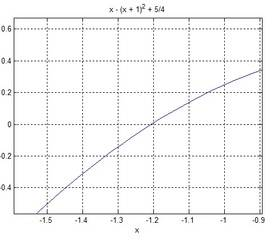I just want to make sure it is done correctly so I can calculate the other integrals, and sketch ##(f*h)(t)## to make sure it is an even symmetric function.

Samy_A
Homework Helper
Thank you for the explanation. Yes ##h## is ##-2t-1## for negative σ. So, for instance for the case ##-1.5 \leq t \leq -1## this is what I found:

$$(f*h)(t) = -\int^{t+1}_{-0.5} h(\sigma) d \sigma = -\int^{t+1}_{-0.5} (-2t-1) \ dt = - \left( (t+1)^2 -(t+1)-0.25 \right)$$

Is this correct?
I find something different.
If I'm not mistaken, your function is equal to -0.5 in -1.5. That should be 0.

Your integral is the same as mine for that case, but the result is different.
When I computed the convolution this morning, I had a number of sign errors. It looks like that's what you have here too.

Last edited:
•roam
I find something different.
If I'm not mistaken, your function is equal to -0.5 in -1.5. That should be 0.

Your integral is the same as mine for that case, but the result is different.
When I computed the convolution this morning, I had a number of sign errors. It looks like that's what you have here too.

Oops, I did the integral again and there was a sign error as you suggested:

$$- \int^{t+1}_{-0.5} (-2t-1).dt = -\Bigg[ \frac{-2 t^2}{2} -t \Bigg]^{t+1}_{-0.5}$$

$$= - \left( \Big[ -(t+1)^2 - (t+1) \Big] - \Big[ -(-0.5)^2 - (-0.5) \Big] \right) = (t+1)^2 + (t+1) + 0.25$$

This time it's a right way up parabola: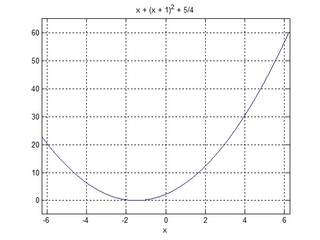Is that what you have also?

Samy_A
Homework Helper
Oops, I did the integral again and there was a sign error as you suggested:

$$- \int^{t+1}_{-0.5} (-2t-1).dt = -\Bigg[ \frac{-2 t^2}{2} -t \Bigg]^{t+1}_{-0.5}$$

$$= - \left( \Big[ -(t+1)^2 - (t+1) \Big] - \Big[ -(-0.5)^2 - (-0.5) \Big] \right) = (t+1)^2 + (t+1) + 0.25$$

This time it's a right way up parabola:Is that what you have also?
Yes, I have the same result. ##(f*h)(t)=t²+3t+\frac{9}{4}## for ##t \in [-1.5, -1]##.
Notice that now the value in -1.5 is 0. The value of the first derivative in -1.5 is also 0. That is as expected, as ##f*h## is 0 below -1.5, and is a differentiable function.

•roam
Thank you so much for the explanation.

For case (c) I found:

$$(f*h)(t)= - \Big[ \int^0_{-0.5} f(t) h(\sigma) d \sigma + \int^{t+1}_{0} f(t) h(\sigma) d \sigma \Big] =- \Big[ \int^0_{-0.5} (-2t-1) d t + \int^{t+1}_{0} (2t-1) d t \Big]$$

$$= -(t+1)^2 + (t+1) + 0.25 = -t^2-t+\frac{1}{4}$$

This function is the inverted version of y=x2+3x+(9/4) we found for part (b).

For case (d) I found:

$$(f*h)(t)= - 2 \int^{0.5}_0 (2t-1)(f(t)=1) dt = 0.5$$

The graph in Matlab looks like this so far for ##t \leq 0## (parts (b), (c), and (d) are shown in magenta, blue and green respectively):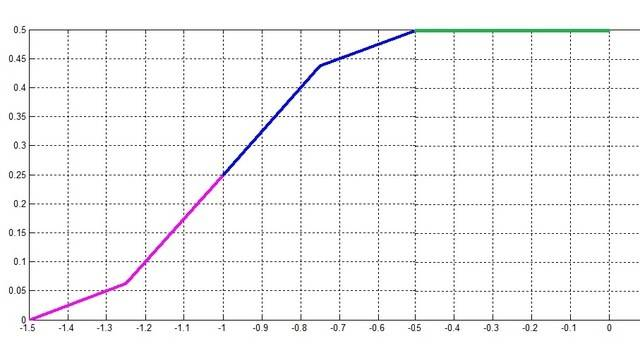Since the ##(f ∗ h)(t)## is continuous and even I think the other half (in blue) should look like this: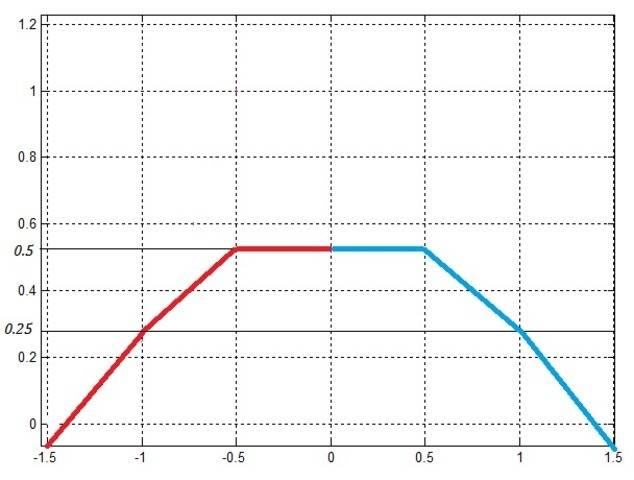Is this correct?

Samy_A
Homework Helper
Yes, I found the same results for cases c) and d).

As ##f*h## is even, you indeed can just take the mirror image of the graph for positive ##t##.
To be clear, from ##-1.5 \to -0.5## the graph segments are parabolic, not straight lines (and likewise on the positive side).

•roam
I get it now. Thank you so much for your time and help.